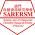### Processing入門與自學指引

Processing是專為互動視覺、創意應用所設計的程式語言，有易於上手的編輯環境、多元函式庫（外掛功能）、豐富的學習資源。語言是基於 Java，但語法結構更為簡單。我們直接用範例來看：

size(500, 500);
line(0, 0, 500, 500);
rect(0, 450, 50, 50);
rect(450, 0, 50, 50);

size(500, 500);
stroke(255, 0, 0); //指定紅色線條。
line(0, 0, 500, 500);
stroke(255, 255, 0); //指定黃色線條。
fill(0, 255, 0); //指定填滿綠色。
rect(0, 450, 50, 50);
fill(0, 0, 255); //指定填滿藍色。
rect(450, 0, 50, 50);

size(500, 500);
for (int i = 0; i < 500; i = i + 10) {
line(0, i, 500, i);
}

// 舞台設定，只會在draw()之前執行一次。
void setup() {
size(500, 500);
}

// 重複繪圖，不斷自動重複執行。
void draw() {
for (int i = 0; i < 500; i = i + 10) {
strokeWeight(i/50); //額外加上的，根據 i 值改變筆劃粗細。
line(0, i, 500, i);
}
}

void setup() {
size(500, 500);
rectMode(CENTER); //改變矩形繪圖方式，以矩形中心為準。
}

void draw() {
fill(random(255), random(255), random(255)); //運用亂數函數產生任意顏色。
rect(mouseX, mouseY, 50, 50);
}

### 留言

1.THANK YOU. 將邀請成為學習processing 的資源